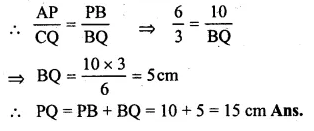Guru

# Question 16. In the given figure, AC is a transverse common tangent to two circles with centres P and Q and of radii 6 cm and 3 cm respectively. Given that AB = 8 cm, calculate PQ.

• 0

This is the basic and conceptual question from Chapter name- circles
Topic – Angle properties of circles
Chapter number- 15

In this question we have that AC is a transverse common tangent to two circles with centres P and Q and of radii 6 cm and 3 cm respectively.

Also Given that AB = 8 cm,

Now we have to calculate PQ.

ICSE Avichal publication
Understanding ICSE Mathematics
Question 16

Share